2019 年 5 月 29 日Seq2Seq 项目地址：

Transformer 项目地址：

## 背景介绍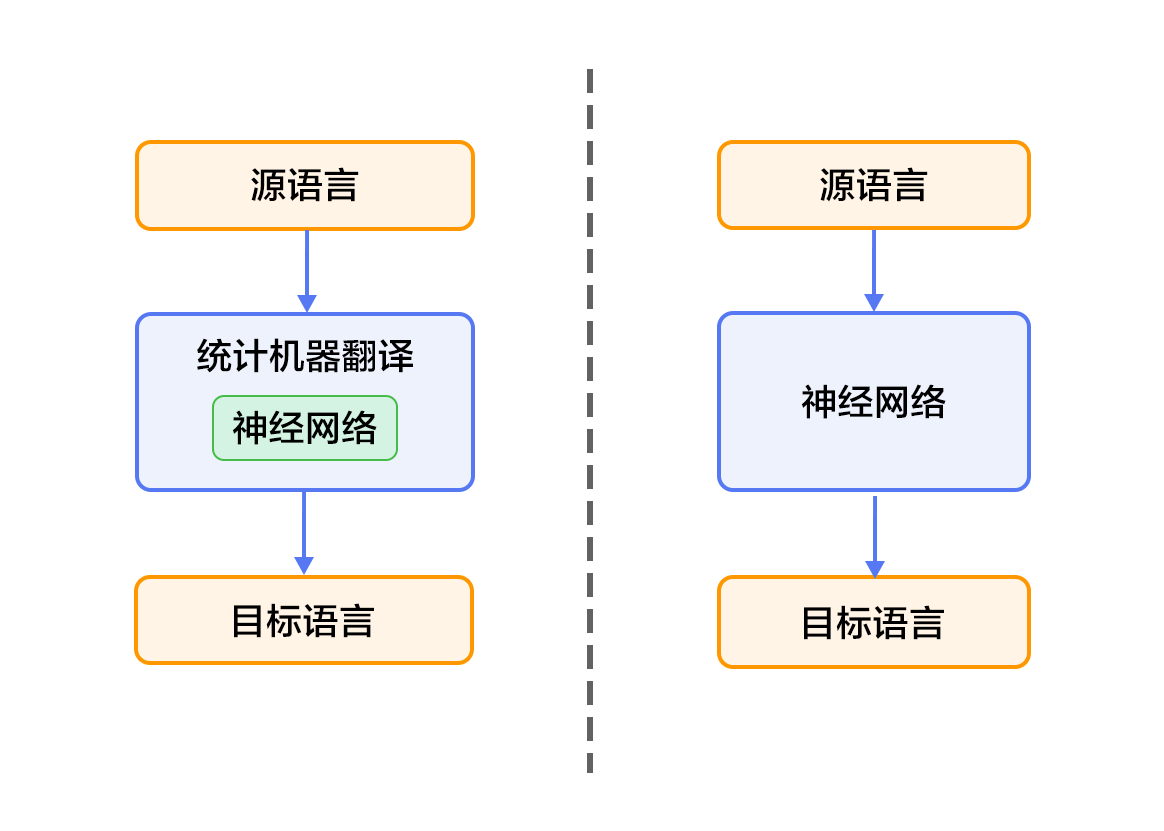## 效果展示

0 -5.36816 These are signs of hope and relief .1 -6.23177 These are the light of hope and relief .2 -7.7914 These are the light of hope and the relief of hope .

• 左起第一列是生成句子的序号；左起第二列是该条句子的得分（从大到小），分值越高越好；左起第三列是生成的英语句子。

• 另外有两个特殊标志：表示句子的结尾，表示未登录词（unknown word），即未在训练字典中出现的词。

## 模型概览

### 双向循环神经网络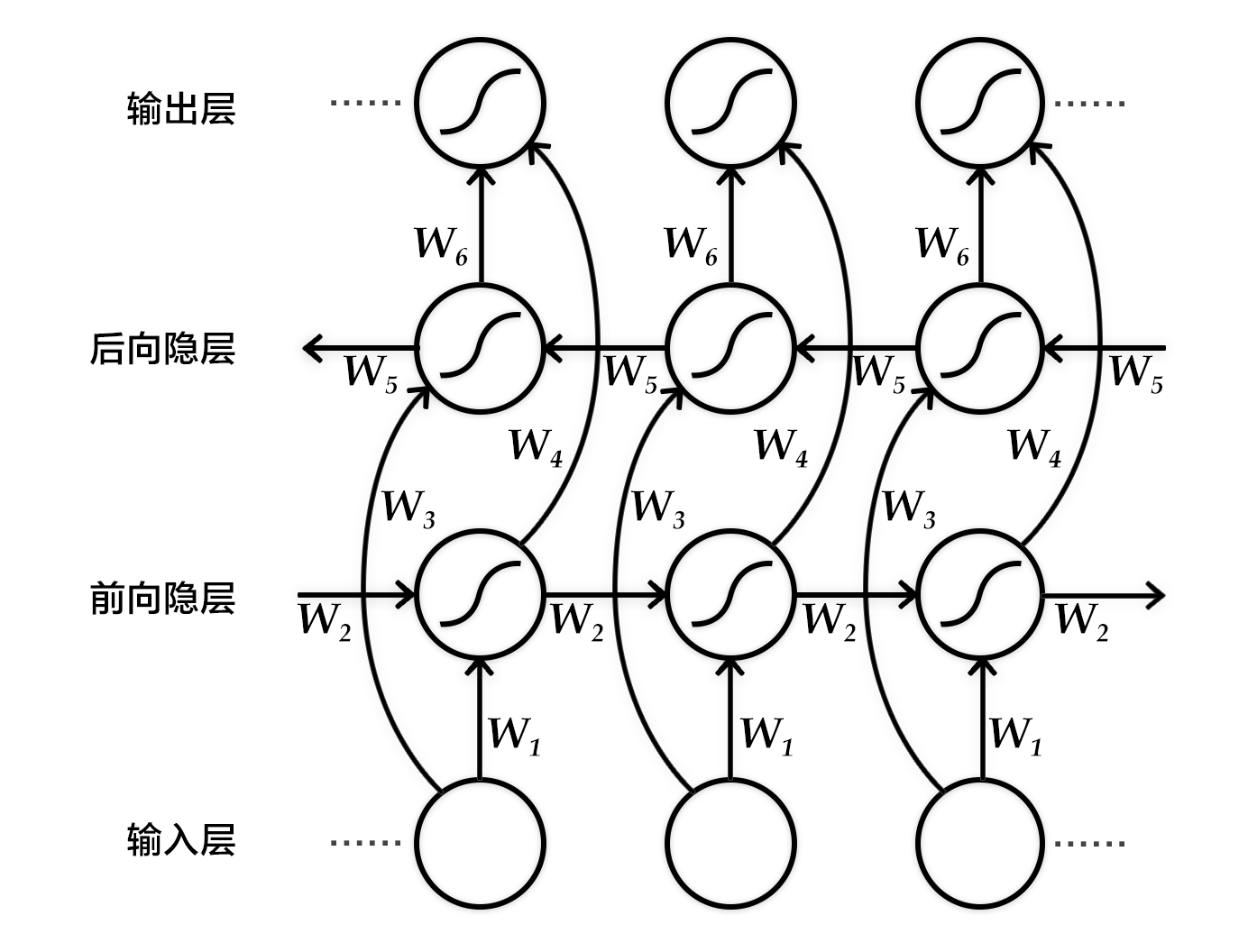### 编码器-解码器框架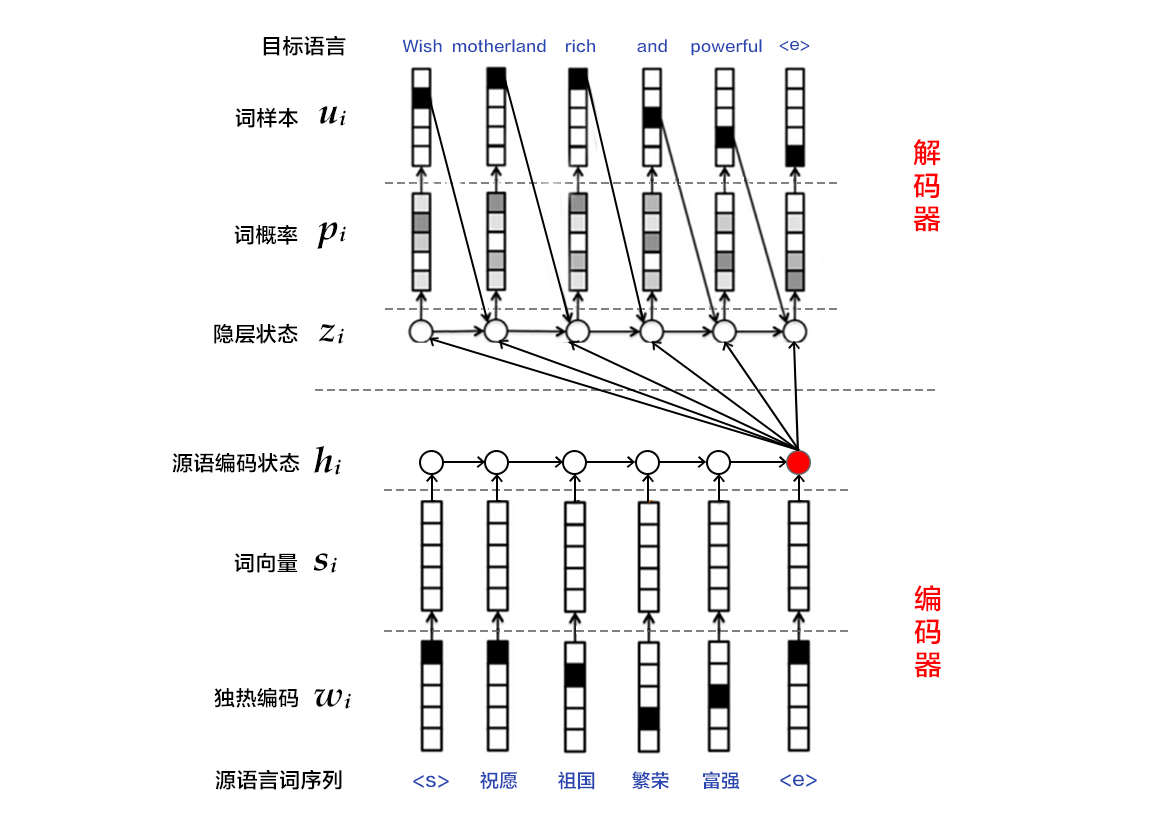### 编码器

1. one-hot vector表示：将源语言句子x={x_1, x_2,…, x_t}的每个词x_i表示成一个列向量W_iϵ〖{0，1}〗^(|v|)，i=1,2,…,T。这个向量W_i的维度与词汇表大小|V| 相同，并且只有一个维度上有值1（该位置对应该词在词汇表中的位置），其余全是0。

2. 映射到低维语义空间的词向量：one-hot vector表示存在两个问题，1）生成的向量维度往往很大，容易造成维数灾难；2）难以刻画词与词之间的关系（如语义相似性，也就是无法很好地表达语义）。因此，需再one-hot vector映射到低维的语义空间，由一个固定维度的稠密向量（称为词向量）表示。记映射矩阵为CϵR^(K*|V|)，用S_i= C_(W_i )表示第i个词的词向量，K为向量维度。

3. 用RNN编码源语言词序列：这一过程的计算公式为h_i = ∅_θ (h_(i-1), S_i)，其中h_0是一个全零的向量，∅_θ是一个非线性激活函数，最后得到的h = {h_1,…, h_T}就是RNN依次读入源语言T个词的状态编码序列。整句话的向量表示可以采用h在最后一个时间步T的状态编码，或使用时间维上的池化（pooling）结果。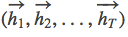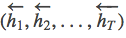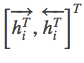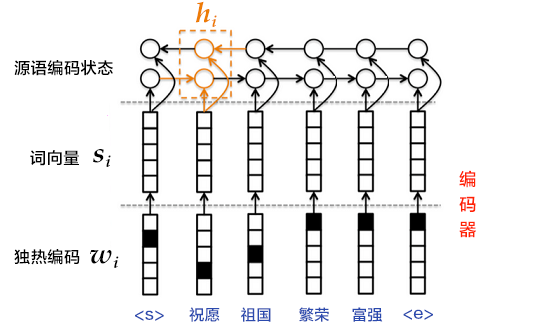### 解码器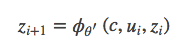1. 将z_(i+1)通过softmax归一化，得到目标语言序列的第i+1个单词的概率分布p_(i+1)。概率分布公式如下：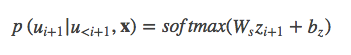2. 根据 p_(i+1)和 u_(i+1)计算代价。

3. 重复步骤 1~23，直到目标语言序列中的所有词处理完毕。

### 柱搜索算法

1. 每一个时刻，根据源语言句子的编码信息 cc、生成的第 ii 个目标语言序列单词 u_i 和 i 时刻 RNN 的隐层状态 z_i，计算出下一个隐层状态 z_(i+1)。

2. 将 z_(i+1)通过 softmax 归一化，得到目标语言序列的第 i+1 个单词的概率分布 p_(i+1)。

3. 根据 p_(i+1)采样出单词 u_(i+1)。

4. 重复步骤 1~3，直到获得句子结束标记或超过句子的最大生成长度为止。

## 数据介绍

### 数据预处理

• 将每个源语言到目标语言的平行语料库文件合并为一个文件：

• 合并每个XXX.src和XXX.trg文件为XXX。

• XXX中的第i行内容为XXX.src中的第i行和XXX.trg中的第i行连接，用’t’分隔。

## 模型配置说明

from __future__ import print_functionimport paddleimport paddle.fluid as fluidimport paddle.fluid.layers as pdimport osimport systry:    from paddle.fluid.contrib.trainer import *    from paddle.fluid.contrib.inferencer import *except ImportError:    print(        "In the fluid 1.0, the trainer and inferencer are moving to paddle.fluid.contrib",        file=sys.stderr)    from paddle.fluid.trainer import *    from paddle.fluid.inferencer import *dict_size = 30000 # 字典维度source_dict_dim = target_dict_dim = dict_size # 源/目标语言字典维度hidden_dim = 32 # 编码器中的隐层大小word_dim = 16 # 词向量维度batch_size = 2 # batch 中的样本数max_length = 8 # 生成句子的最大长度topk_size = 50beam_size = 2 # 柱宽度is_sparse = Truedecoder_size = hidden_dim # 解码器中的隐层大小model_save_dir = "machine_translation.inference.model"

def encoder(is_sparse): # 定义源语言id序列的输入数据 src_word_id = pd.data(     name="src_word_id", shape=, dtype='int64', lod_level=1) # 将上述编码映射到低维语言空间的词向量 src_embedding = pd.embedding(     input=src_word_id,     size=[dict_size, word_dim],     dtype='float32',     is_sparse=is_sparse,     param_attr=fluid.ParamAttr(name='vemb')) # LSTM层：fc + dynamic_lstm fc1 = pd.fc(input=src_embedding, size=hidden_dim * 4, act='tanh') lstm_hidden0, lstm_0 = pd.dynamic_lstm(input=fc1, size=hidden_dim * 4) # 取源语言序列编码后的最后一个状态 encoder_out = pd.sequence_last_step(input=lstm_hidden0) return encoder_out

def train_decoder(context):    # 定义目标语言id序列的输入数据，并映射到低维语言空间的词向量    trg_language_word = pd.data(        name="target_language_word", shape=, dtype='int64', lod_level=1)    trg_embedding = pd.embedding(        input=trg_language_word,        size=[dict_size, word_dim],        dtype='float32',        is_sparse=is_sparse,        param_attr=fluid.ParamAttr(name='vemb'))    rnn = pd.DynamicRNN()    with rnn.block(): # 使用 DynamicRNN 定义每一步的计算        # 获取当前步目标语言输入的词向量        current_word = rnn.step_input(trg_embedding)        # 获取隐层状态        pre_state = rnn.memory(init=context)        # 解码器计算单元：单层前馈网络        current_state = pd.fc(input=[current_word, pre_state],                              size=decoder_size,                              act='tanh')        # 计算归一化的单词预测概率        current_score = pd.fc(input=current_state,                              size=target_dict_dim,                              act='softmax')        # 更新RNN的隐层状态        rnn.update_memory(pre_state, current_state)        # 输出预测概率        rnn.output(current_score)    return rnn()

def decode(context):    init_state = context    # 定义解码过程循环计数变量    array_len = pd.fill_constant(shape=, dtype='int64', value=max_length)    counter = pd.zeros(shape=, dtype='int64', force_cpu=True)    # 定义 tensor array 用以保存各个时间步的内容，并写入初始id，score和state    state_array = pd.create_array('float32')    pd.array_write(init_state, array=state_array, i=counter)    ids_array = pd.create_array('int64')    scores_array = pd.create_array('float32')    init_ids = pd.data(name="init_ids", shape=, dtype="int64", lod_level=2)    init_scores = pd.data(        name="init_scores", shape=, dtype="float32", lod_level=2)    pd.array_write(init_ids, array=ids_array, i=counter)    pd.array_write(init_scores, array=scores_array, i=counter)    # 定义循环终止条件变量    cond = pd.less_than(x=counter, y=array_len)    # 定义 while_op    while_op = pd.While(cond=cond)    with while_op.block(): # 定义每一步的计算        # 获取解码器在当前步的输入，包括上一步选择的id，对应的score和上一步的state        pre_ids = pd.array_read(array=ids_array, i=counter)        pre_state = pd.array_read(array=state_array, i=counter)        pre_score = pd.array_read(array=scores_array, i=counter)        # 更新输入的state为上一步选择id对应的state        pre_state_expanded = pd.sequence_expand(pre_state, pre_score)        # 同训练模式下解码器中的计算逻辑，包括获取输入向量，解码器计算单元计算和        # 归一化单词预测概率的计算        pre_ids_emb = pd.embedding(            input=pre_ids,            size=[dict_size, word_dim],            dtype='float32',            is_sparse=is_sparse，  param_attr=fluid.ParamAttr(name='vemb'))        current_state = pd.fc(input=[pre_state_expanded, pre_ids_emb],                              size=decoder_size,                              act='tanh')        current_state_with_lod = pd.lod_reset(x=current_state, y=pre_score)        current_score = pd.fc(input=current_state_with_lod,                              size=target_dict_dim,                              act='softmax')        topk_scores, topk_indices = pd.topk(current_score, k=beam_size)        # 计算累计得分，进行beam search        accu_scores = pd.elementwise_add(            x=pd.log(topk_scores), y=pd.reshape(pre_score, shape=[-1]), axis=0)        selected_ids, selected_scores = pd.beam_search(            pre_ids,            pre_score,            topk_indices,            accu_scores,            beam_size,            end_id=10,            level=0)    with pd.Switch() as switch:            with switch.case(pd.is_empty(selected_ids)):                pd.fill_constant(                    shape=, value=0, dtype='bool', force_cpu=True, out=cond)            with switch.default():                pd.increment(x=counter, value=1, in_place=True)                pd.array_write(current_state, array=state_array, i=counter)                pd.array_write(selected_ids, array=ids_array, i=counter)                pd.array_write(selected_scores, array=scores_array, i=counter)                length_cond = pd.less_than(x=counter, y=array_len)                finish_cond = pd.logical_not(pd.is_empty(x=selected_ids))                pd.logical_and(x=length_cond, y=finish_cond, out=cond)    translation_ids, translation_scores = pd.beam_search_decode(        ids=ids_array, scores=scores_array, beam_size=beam_size, end_id=10)    return translation_ids, translation_scores

def train_program():    context = encoder()    rnn_out = train_decoder(context)    label = pd.data(        name="target_language_next_word", shape=, dtype='int64', lod_level=1)    cost = pd.cross_entropy(input=rnn_out, label=label)    avg_cost = pd.mean(cost)    return avg_costdef optimizer_func():    return fluid.optimizer.Adagrad(        learning_rate=1e-4,        regularization=fluid.regularizer.L2DecayRegularizer(            regularization_coeff=0.1))

## 训练模型

### 定义训练环境

if use_cuda and not fluid.core.is_compiled_with_cuda():  returnplace = fluid.CUDAPlace(0) if use_cuda else fluid.CPUPlace()

### 定义数据提供器

train_reader = paddle.batch(        paddle.reader.shuffle(            paddle.dataset.wmt14.train(dict_size), buf_size=1000),        batch_size=batch_size)

### 构造训练器(trainer)

trainer = Trainer(        train_func=train_program, place=place, optimizer_func=optimizer_func)

### 提供数据

feed_order 用来定义每条产生的数据和 paddle.layer.data 之间的映射关系。比如，wmt14.train 产生的第一列的数据对应的是 src_word_id 这个特征。

feed_order = [        'src_word_id', 'target_language_word', 'target_language_next_word'    ]

### 事件处理器

def event_handler(event):    if isinstance(event, EndStepEvent):        if event.step % 10 == 0:            print('pass_id=' + str(event.epoch) + ' batch=' + str(event.step))  if isinstance(event, EndEpochEvent):    trainer.save_params(model_save_dir)

### 开始训练

trainer = Trainer(        train_func=train_program, place=place, optimizer_func=optimizer_func)trainer.train(        reader=train_reader,        num_epochs=EPOCH_NUM,        event_handler=event_handler,        feed_order=feed_order)

## 应用模型

### 定义解码部分

context = encoder()translation_ids, translation_scores = decode(context)

### 定义数据

init_ids_data = np.array([1 for _ in range(batch_size)], dtype='int64')init_scores_data = np.array(    [1. for _ in range(batch_size)], dtype='float32')init_ids_data = init_ids_data.reshape((batch_size, 1))init_scores_data = init_scores_data.reshape((batch_size, 1))init_lod =  * batch_sizeinit_lod = [init_lod, init_lod]init_ids = fluid.create_lod_tensor(init_ids_data, init_lod, place)init_scores = fluid.create_lod_tensor(init_scores_data, init_lod, place)test_data = paddle.batch(    paddle.reader.shuffle(        paddle.dataset.wmt14.test(dict_size), buf_size=1000),    batch_size=batch_size)feed_order = ['src_word_id']feed_list = [    framework.default_main_program().global_block().var(var_name)    for var_name in feed_order]feeder = fluid.DataFeeder(feed_list, place)src_dict, trg_dict = paddle.dataset.wmt14.get_dict(dict_size)

### 测试

for data in test_data():    feed_data = map(lambda x: [x], data)    feed_dict = feeder.feed(feed_data)    feed_dict['init_ids'] = init_ids    feed_dict['init_scores'] = init_scores    results = exe.run(        framework.default_main_program(),        feed=feed_dict,        fetch_list=[translation_ids, translation_scores],        return_numpy=False)    result_ids = np.array(results)    result_ids_lod = results.lod()    result_scores = np.array(results)    print("Original sentence:")    print(" ".join([src_dict[w] for w in feed_data[1:-1]]))    print("Translated score and sentence:")    for i in xrange(beam_size):        start_pos = result_ids_lod[i] + 1        end_pos = result_ids_lod[i+1]        print("%d\t%.4f\t%s\n" % (i+1, result_scores[end_pos-1],                " ".join([trg_dict[w] for w in result_ids[start_pos:end_pos]])))    break

## 参考文献

2. Cho K, Van Merriënboer B, Gulcehre C, et al. Learning phrase representations using RNN encoder-decoder for statistical machine translation[C]//Proceedings of the 2014 Conference on Empirical Methods in Natural Language Processing (EMNLP), 2014: 1724-1734.

3. Chung J, Gulcehre C, Cho K H, et al. Empirical evaluation of gated recurrent neural networks on sequence modeling[J]. arXiv preprint arXiv:1412.3555, 2014.

4. Bahdanau D, Cho K, Bengio Y. Neural machine translation by jointly learning to align and translate[C]//Proceedings of ICLR 2015, 2015.

5. Papineni K, Roukos S, Ward T, et al. BLEU: a method for automatic evaluation of machine translation[C]//Proceedings of the 40th annual meeting on association for computational linguistics. Association for Computational Linguistics, 2002: 311-318.

2019 年 5 月 29 日 15:326057

## 评论

• ###### 深度学习利器：TensorFlow 与 NLP 模型

传统的自然语言处理方法涉及到了很多语言学本身的知识，而深度学习，是表征学习（representation learning）的一种方法，在机器翻译、自动问答、文本分类、情感分析、信息抽取、序列标注、语法解析等领域都有广泛的应用。本文作者将主要结合 TensorFlow 平台，讲解 TensorFlow 词向量生成模型（Vector Representations of Words）；使用 RNN、LSTM 模型进行语言预测；以及 TensorFlow 自动翻译模型。

• ###### 基函数扩展：属性的非线性化

要获得更强的表达能力，必须要把非线性的元素纳入到机器学习模型之中。今天我们就聊聊通过基函数扩展实现非线性模型的方法。

2018 年 7 月 21 日

• ###### 关于机器翻译，看这一篇就够了

本文转载自公众号携程技术（ID：ctriptech）。

• ###### 29 丨 EM 聚类（下）：用 EM 算法对王者荣耀英雄进行划分

如何使用EM算法工具完成聚类？

2019 年 2 月 18 日

• ###### 集成化处理：Boosting 与 Bagging

集成学习的作用就是将这多个弱学习器提升成一个强学习器。今天我们就来聊聊集成学习的基本原理，以及典型的集成学习方法。

2018 年 8 月 2 日

• ###### 深入浅出 TensorFlow（二）：TensorFlow 解决 MNIST 问题入门

本文是整个系列的第二篇文章，将会简单介绍TensorFlow安装方法、TensorFlow基本概念、神经网络基本模型，并在MNIST数据集上使用TensorFlow实现一个简单的神经网络。

• ###### NLP 中的预训练语言模型总结

本文以QA形式总结对比了nlp中的预训练语言模型。

• ###### 上交大提出支持并行计算的 SRNN：比 RNN 快 136 倍！（代码已开源）

本文介绍了切片循环神经网络，可以通过将序列分割成多个子序列来实现并行化计算。SRNN具有通过多层网络和极少的额外参数来获得高层次信息的能力。不需要改变循环单元，SRNN的速度便可以达到标准RNN的136倍，而且训练更长的序列时，SRNN的速度能够更快。作者在六个大规模情感分析数据集上进行了实验，实验结果表明SRNN比标准的RNN具有更好的性能。

• ###### 特征处理：如何利用 Spark 解决特征处理问题？

像动作、喜剧、爱情、科幻这些电影风格，是怎么转换成数值供推荐模型使用的呢？用户的行为历史又是怎么转换成数值特征的呢？

2020 年 10 月 12 日

• ###### 与其感慨模型难训练，不如试试 AutoNLP

你或许知道AutoML，但未必用过AutoNLP。

• ###### 五问 BERT：深入理解 NLP 领域爆红的预训练模型

本文由浅入深，通过理论与案例多角度介绍了BERT的理论与使用方法。

• ###### Embedding+MLP：如何用 TensorFlow 实现经典的深度学习模型？

你知道微软在2016年提出的深度学习模型Deep Crossing吗？它就属于经典的Embedding+MLP模型。

2020 年 11 月 18 日

• ###### LDA 模型的前世今生

今天我要介绍的是LDA的模型，它在过去十年里开启了一个领域叫主题模型。一段时间内，LDA是分析文本信息的标准工具。

2017 年 10 月 18 日

• ###### 招商证券 BERT 压缩实践：如何大幅提高模型推断速度？

BERT虽然强大，但也并非全能。为了使它更符合工程需求，我们要给BERT“减减肥”。

• ###### Facebook 通过 10 亿单词构建有效的神经网络语言模型

FAIR开发并开源了一个名为torch-rnnlib 的类库，该类库允许研究者设计新的递归模型，并以最小的努力在GPU上测试这些原型。最近Edouard Grave、Justin Chiu 和Armand Joulin在Facebook的网站上发表了一篇文章，介绍了用户如何通过该类库设计新的递归网络。

• ###### 谷歌最强 NLP 预训练模型 BERT 正式开源

近日，谷歌AI的一篇NLP论文引起了社区极大的关注与讨论，被认为是NLP领域的极大突破。该论文介绍了一种新的语言表征模型BERT——来自Transformer的双向编码器表征。

• ###### 谷歌开源先进语言模型 Transformer-XL：集 Transformer 和 RNN 之大成

与最先进Transformer模型相比，推理速度能提高300-1800倍

• ###### TensorFlow 工程实战（四）：使用带注意力机制的模型分析评论者是否满意

如何利用tf.keras接口搭建一个只带有注意力机制的模型，进而实现文本分类？

spring

#### 奈学教育《百万架构师》课程大纲（1）